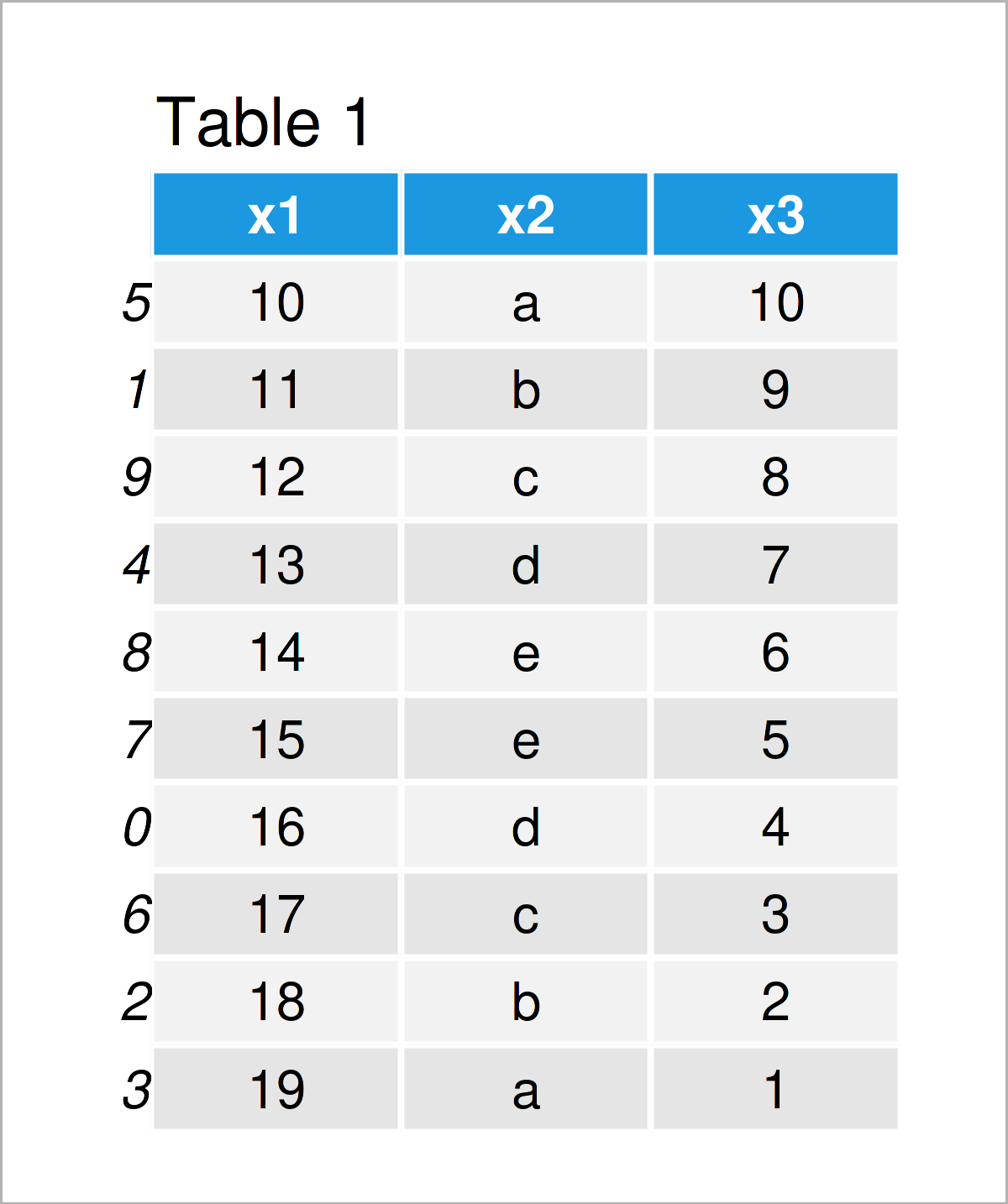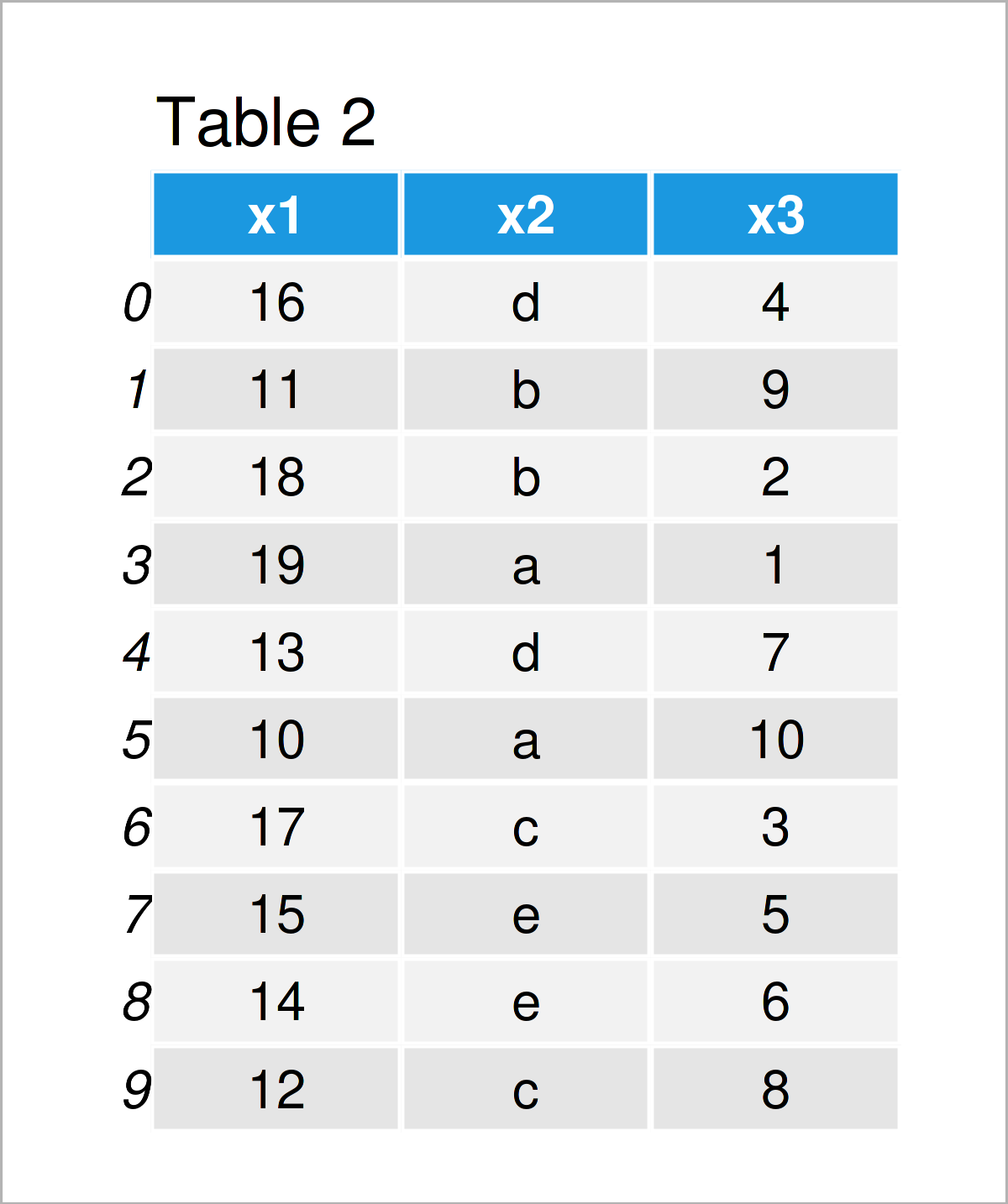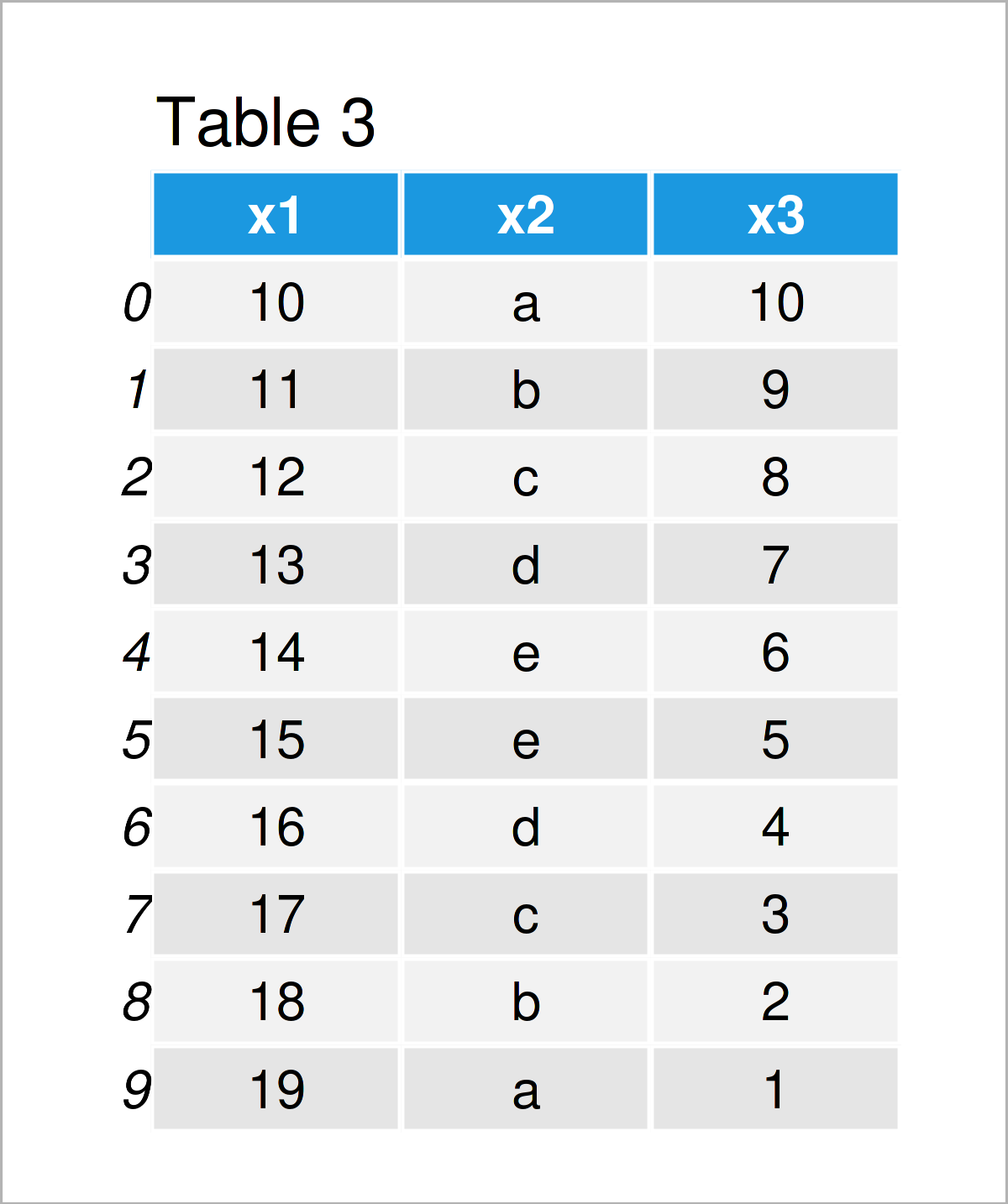# Sort Index of pandas DataFrame in Python (2 Examples)

In this Python programming article you’ll learn how to order the rows of a pandas DataFrame by index.

The page consists of the following content:

Let’s dig in!

## Example Data & Libraries

First, we need to import the pandas library:

`import pandas as pd                          # Import pandas library to Python`

We use the following data as a basis for this Python programming tutorial:

```data = pd.DataFrame({'x1':range(10, 20),     # Create pandas DataFrame
'x2':['a', 'b', 'c', 'd', 'e', 'e', 'd', 'c', 'b', 'a'],
'x3':range(10, 0, - 1)},
index = [5, 1, 9, 4, 8, 7, 0, 6, 2, 3])
print(data)                                  # Print pandas DataFrame```Have a look at the previous table. It shows that the exemplifying pandas DataFrame has ten rows and three columns. You can also see that the indices of this data set are not ordered.

## Example 1: Order Rows of pandas DataFrame by Index Using sort_index() Function

Example 1 illustrates how to reorder the rows of a pandas DataFrame based on the index of this DataFrame.

For this task, we can apply the sort_index function as shown in the following Python code:

```data_new1 = data.sort_index()                # Apply sort_index function
print(data_new1)                             # Print updated DataFrame```By running the previously shown Python programming syntax, we have created Table 2, i.e. a new pandas DataFrame where the rows have been sorted according to the index of this DataFrame.

Note that the values within the DataFrame have also been rearranged. In the next example, I’ll show how to reset the indices of a pandas DataFrame without moving the actual values in this DataFrame.

Let’s do this!

## Example 2: Reset Index of pandas DataFrame Using reset_index() Function

Example 2 illustrates how to change the index numbers to a range from 0 to the number of rows without changing the order of the values in this DataFrame.

To achieve this, we can apply the reset_index function as illustrated below:

```data_new2 = data.reset_index(drop = True)    # Apply reset_index function
print(data_new2)                             # Print updated DataFrame```By executing the previous Python code, we have created Table 3, i.e. another pandas DataFrame. This data set contains the same order of the values in the data cells. However, the index numbers have been reset.

## Video, Further Resources & Summary

Do you need more info on the Python codes of this tutorial? Then I recommend watching the following video instruction on my YouTube channel. In the video, I’m explaining the content of this tutorial in a live session:

Please accept YouTube cookies to play this video. By accepting you will be accessing content from YouTube, a service provided by an external third party.If you accept this notice, your choice will be saved and the page will refresh.

In addition, you may have a look at the other articles on this website. I have released numerous tutorials already.

To summarize: You have learned on this page how to sort and reindex the rows of a pandas DataFrame by index in the Python programming language. Don’t hesitate to let me know in the comments, in case you have additional questions.

Subscribe to the Statistics Globe Newsletter